Скачать презентацию International Conference The Many Dimension of Poverty Brasilia

d9c64a2cdedfa89c66242a92a5e2c5f8.ppt

• Количество слайдов: 29International Conference The Many Dimension of Poverty Brasilia, Brazil – 29 -31 August 2005 1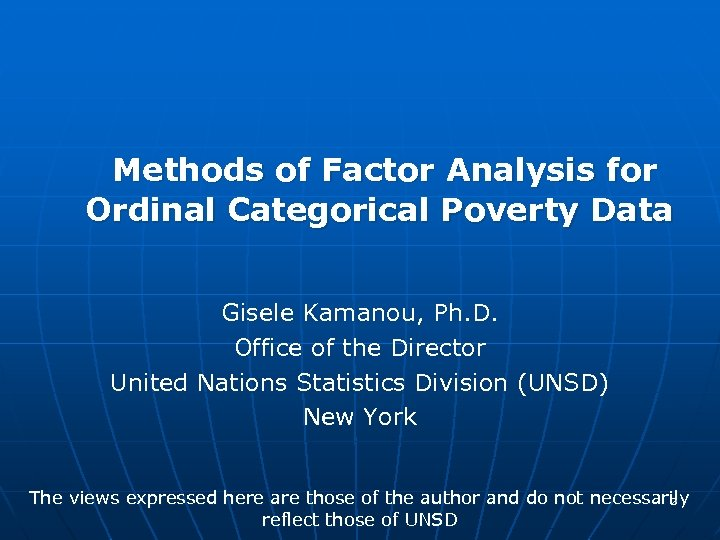Methods of Factor Analysis for Ordinal Categorical Poverty Data Gisele Kamanou, Ph. D. Office of the Director United Nations Statistics Division (UNSD) New York The views expressed here are those of the author and do not necessarily 2 reflect those of UNSD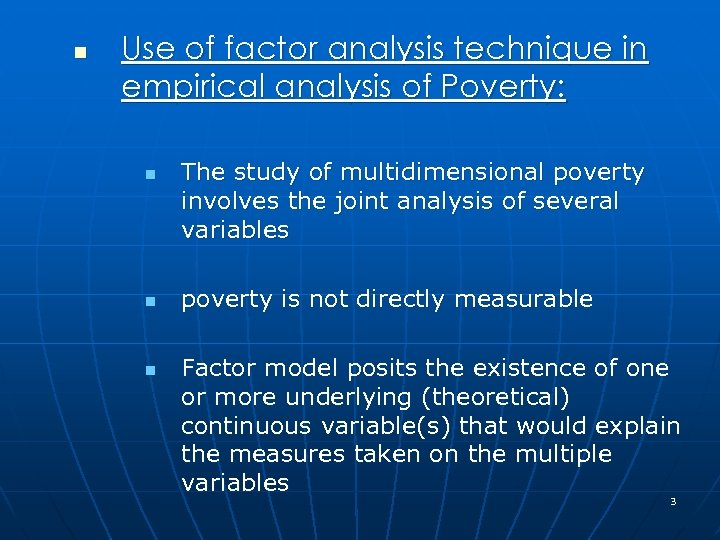n Use of factor analysis technique in empirical analysis of Poverty: n n n The study of multidimensional poverty involves the joint analysis of several variables poverty is not directly measurable Factor model posits the existence of one or more underlying (theoretical) continuous variable(s) that would explain the measures taken on the multiple variables 3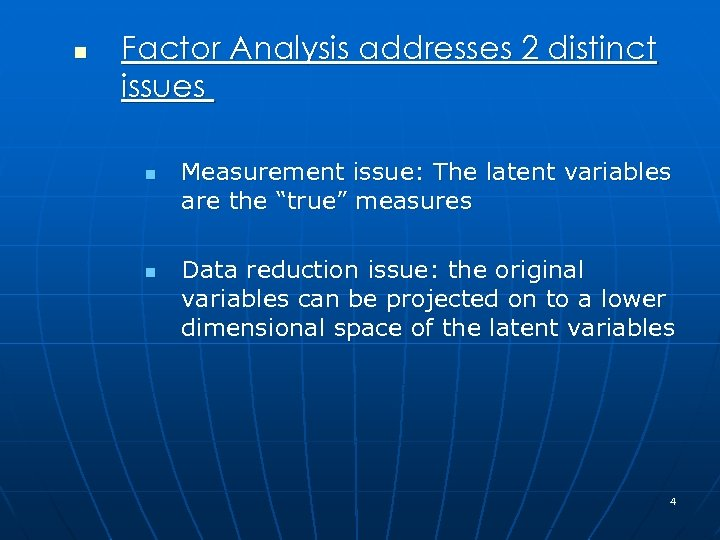n Factor Analysis addresses 2 distinct issues n n Measurement issue: The latent variables are the “true” measures Data reduction issue: the original variables can be projected on to a lower dimensional space of the latent variables 4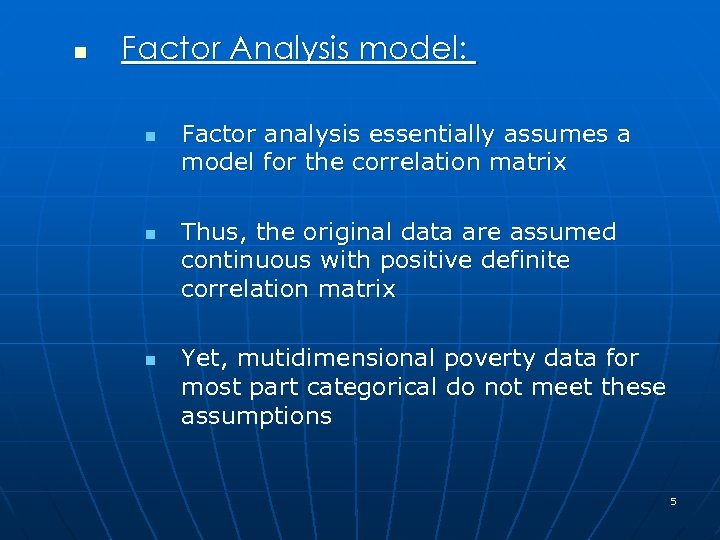n Factor Analysis model: n n n Factor analysis essentially assumes a model for the correlation matrix Thus, the original data are assumed continuous with positive definite correlation matrix Yet, mutidimensional poverty data for most part categorical do not meet these assumptions 5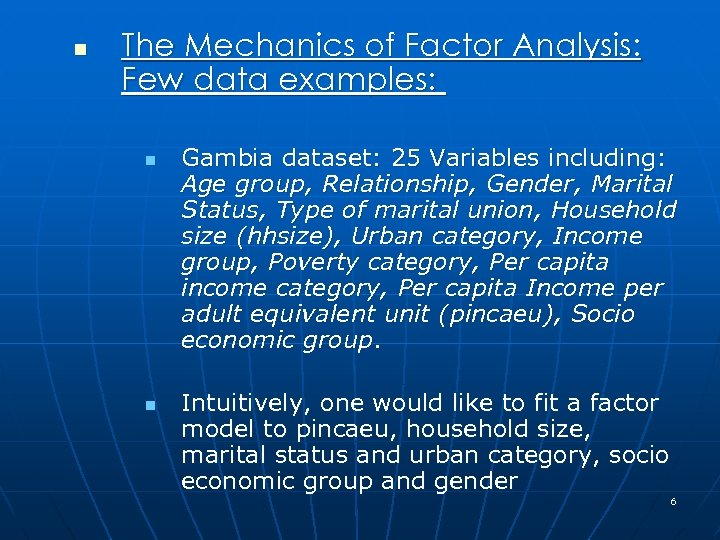n The Mechanics of Factor Analysis: Few data examples: n n Gambia dataset: 25 Variables including: Age group, Relationship, Gender, Marital Status, Type of marital union, Household size (hhsize), Urban category, Income group, Poverty category, Per capita income category, Per capita Income per adult equivalent unit (pincaeu), Socio economic group. Intuitively, one would like to fit a factor model to pincaeu, household size, marital status and urban category, socio economic group and gender 6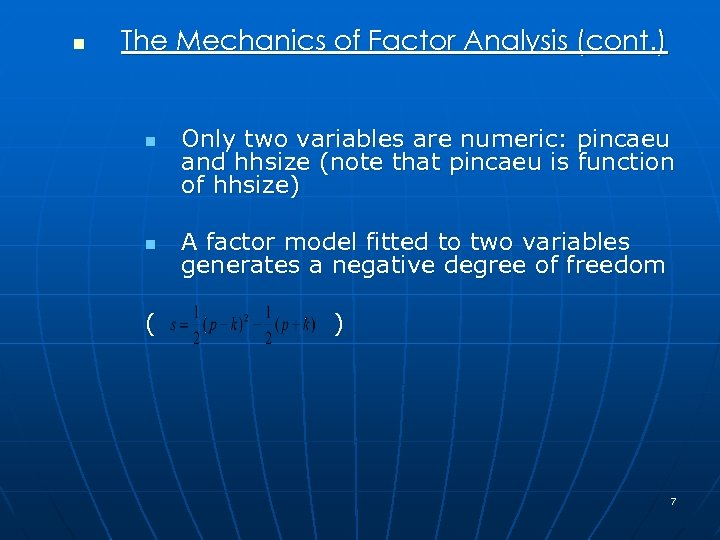n The Mechanics of Factor Analysis (cont. ) n n ( Only two variables are numeric: pincaeu and hhsize (note that pincaeu is function of hhsize) A factor model fitted to two variables generates a negative degree of freedom ) 7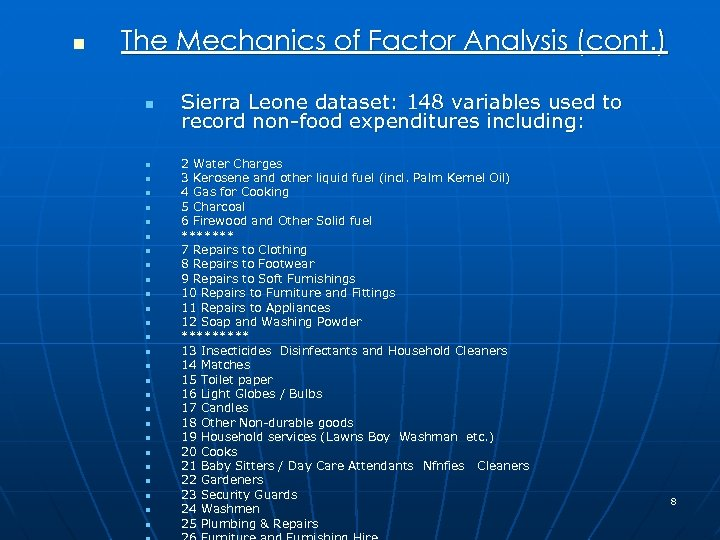n The Mechanics of Factor Analysis (cont. ) n n n n n n n Sierra Leone dataset: 148 variables used to record non-food expenditures including: 2 Water Charges 3 Kerosene and other liquid fuel (incl. Palm Kernel Oil) 4 Gas for Cooking 5 Charcoal 6 Firewood and Other Solid fuel ******* 7 Repairs to Clothing 8 Repairs to Footwear 9 Repairs to Soft Furnishings 10 Repairs to Furniture and Fittings 11 Repairs to Appliances 12 Soap and Washing Powder ***** 13 Insecticides Disinfectants and Household Cleaners 14 Matches 15 Toilet paper 16 Light Globes / Bulbs 17 Candles 18 Other Non-durable goods 19 Household services (Lawns Boy Washman etc. ) 20 Cooks 21 Baby Sitters / Day Care Attendants Nfnfies Cleaners 22 Gardeners 23 Security Guards 24 Washmen 25 Plumbing & Repairs 8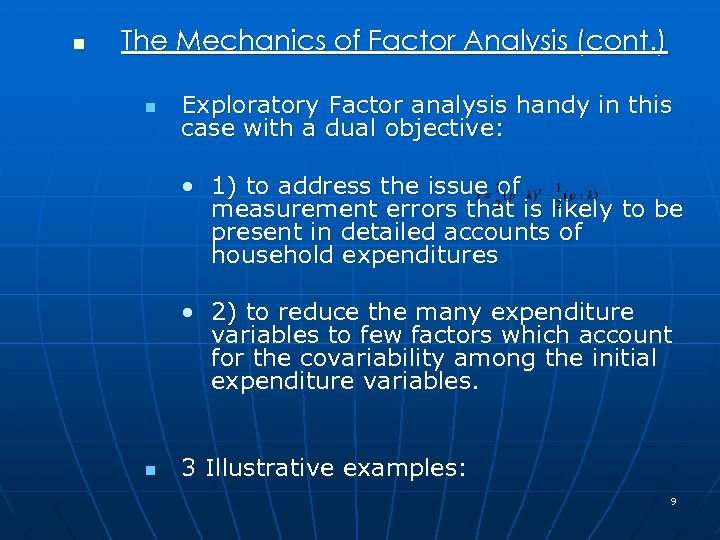n The Mechanics of Factor Analysis (cont. ) n Exploratory Factor analysis handy in this case with a dual objective: • 1) to address the issue of measurement errors that is likely to be present in detailed accounts of household expenditures • 2) to reduce the many expenditure variables to few factors which account for the covariability among the initial expenditure variables. n 3 Illustrative examples: 9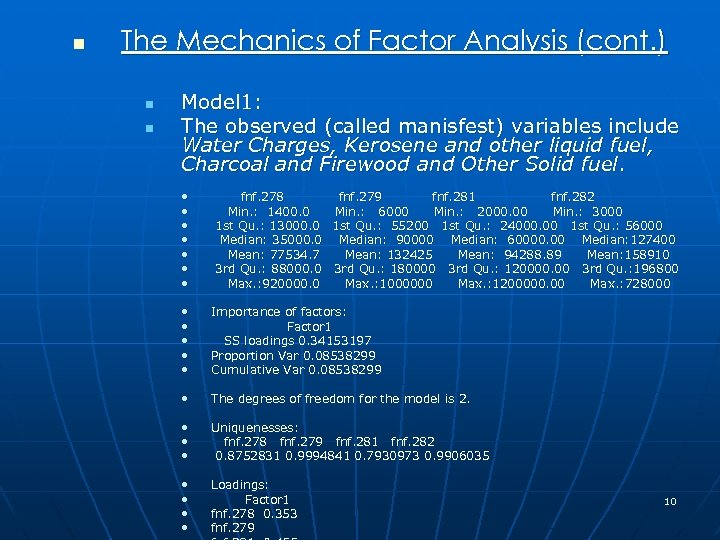n The Mechanics of Factor Analysis (cont. ) n n Model 1: The observed (called manisfest) variables include Water Charges, Kerosene and other liquid fuel, Charcoal and Firewood and Other Solid fuel. • • fnf. 278 fnf. 279 fnf. 281 fnf. 282 Min. : 1400. 0 Min. : 6000 Min. : 2000. 00 Min. : 3000 1 st Qu. : 13000. 0 1 st Qu. : 55200 1 st Qu. : 24000. 00 1 st Qu. : 56000 Median: 35000. 0 Median: 90000 Median: 60000. 00 Median: 127400 Mean: 77534. 7 Mean: 132425 Mean: 94288. 89 Mean: 158910 3 rd Qu. : 88000. 0 3 rd Qu. : 180000 3 rd Qu. : 120000. 00 3 rd Qu. : 196800 Max. : 920000. 0 Max. : 1000000 Max. : 1200000. 00 Max. : 728000 • • • Importance of factors: Factor 1 SS loadings 0. 34153197 Proportion Var 0. 08538299 Cumulative Var 0. 08538299 • The degrees of freedom for the model is 2. • • • Uniquenesses: fnf. 278 fnf. 279 fnf. 281 fnf. 282 0. 8752831 0. 9994841 0. 7930973 0. 9906035 • • Loadings: Factor 1 fnf. 278 0. 353 fnf. 279 10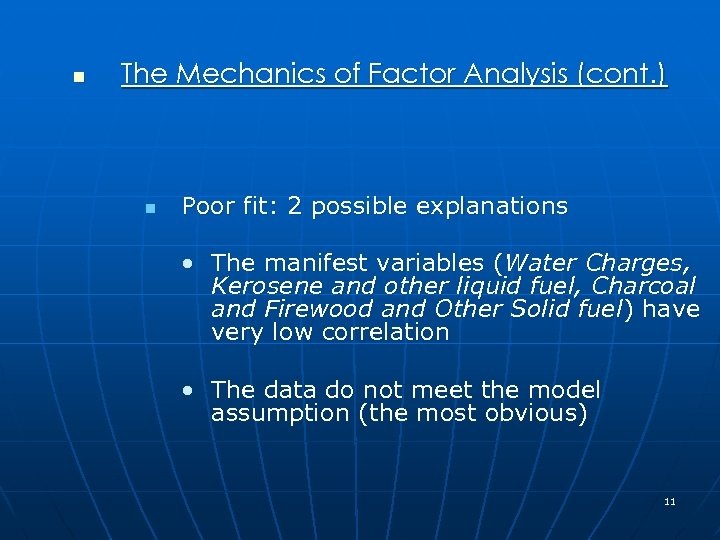n The Mechanics of Factor Analysis (cont. ) n Poor fit: 2 possible explanations • The manifest variables (Water Charges, Kerosene and other liquid fuel, Charcoal and Firewood and Other Solid fuel) have very low correlation • The data do not meet the model assumption (the most obvious) 11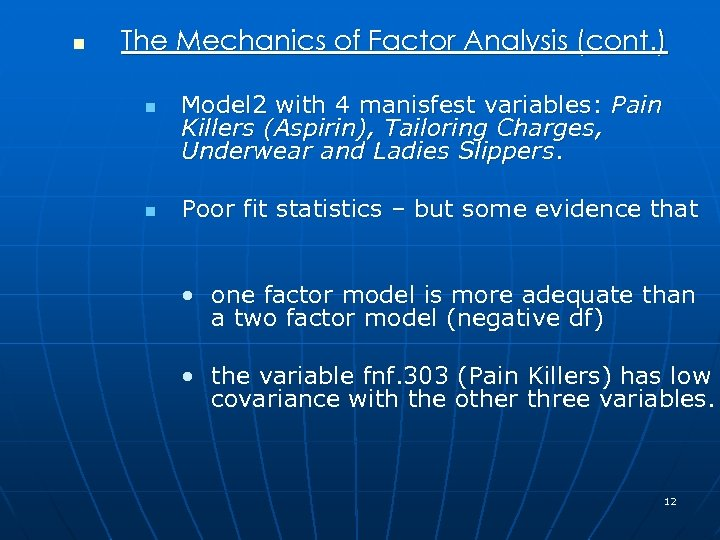n The Mechanics of Factor Analysis (cont. ) n n Model 2 with 4 manisfest variables: Pain Killers (Aspirin), Tailoring Charges, Underwear and Ladies Slippers. Poor fit statistics – but some evidence that • one factor model is more adequate than a two factor model (negative df) • the variable fnf. 303 (Pain Killers) has low covariance with the other three variables. 12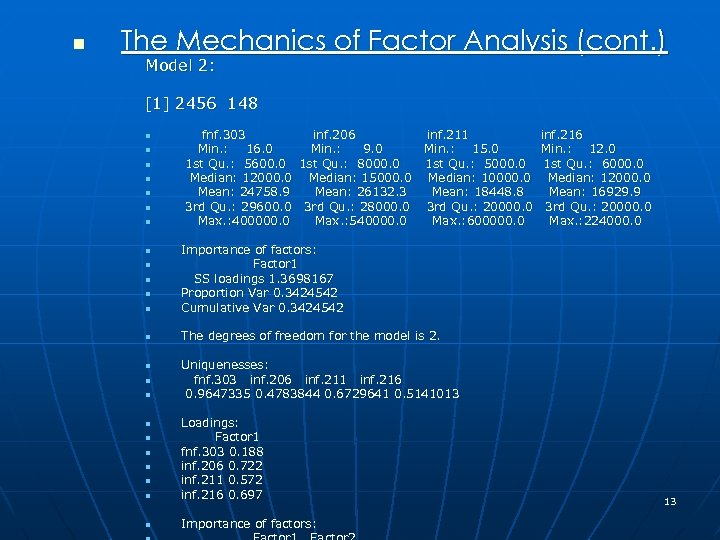n The Mechanics of Factor Analysis (cont. ) Model 2:  2456 148 n n n n fnf. 303 inf. 206 Min. : 16. 0 Min. : 9. 0 1 st Qu. : 5600. 0 1 st Qu. : 8000. 0 Median: 12000. 0 Median: 15000. 0 Mean: 24758. 9 Mean: 26132. 3 3 rd Qu. : 29600. 0 3 rd Qu. : 28000. 0 Max. : 400000. 0 Max. : 540000. 0 inf. 211 Min. : 15. 0 1 st Qu. : 5000. 0 Median: 10000. 0 Mean: 18448. 8 3 rd Qu. : 20000. 0 Max. : 600000. 0 n Importance of factors: Factor 1 SS loadings 1. 3698167 Proportion Var 0. 3424542 Cumulative Var 0. 3424542 n inf. 216 Min. : 12. 0 1 st Qu. : 6000. 0 Median: 12000. 0 Mean: 16929. 9 3 rd Qu. : 20000. 0 Max. : 224000. 0 The degrees of freedom for the model is 2. n n n n Uniquenesses: fnf. 303 inf. 206 inf. 211 inf. 216 0. 9647335 0. 4783844 0. 6729641 0. 5141013 n Loadings: Factor 1 fnf. 303 0. 188 inf. 206 0. 722 inf. 211 0. 572 inf. 216 0. 697 n Importance of factors: n n n 13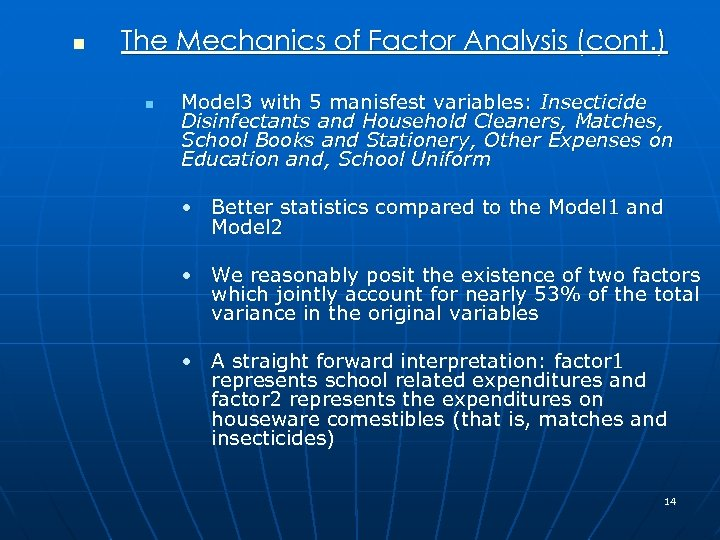n The Mechanics of Factor Analysis (cont. ) n Model 3 with 5 manisfest variables: Insecticide Disinfectants and Household Cleaners, Matches, School Books and Stationery, Other Expenses on Education and, School Uniform • Better statistics compared to the Model 1 and Model 2 • We reasonably posit the existence of two factors which jointly account for nearly 53% of the total variance in the original variables • A straight forward interpretation: factor 1 represents school related expenditures and factor 2 represents the expenditures on houseware comestibles (that is, matches and insecticides) 14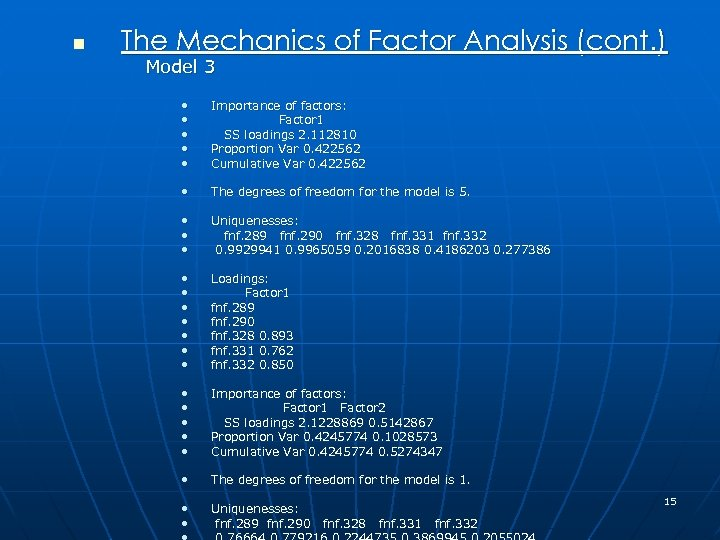n The Mechanics of Factor Analysis (cont. ) Model 3 • • • Importance of factors: Factor 1 SS loadings 2. 112810 Proportion Var 0. 422562 Cumulative Var 0. 422562 • The degrees of freedom for the model is 5. • • • Uniquenesses: fnf. 289 fnf. 290 fnf. 328 fnf. 331 fnf. 332 0. 9929941 0. 9965059 0. 2016838 0. 4186203 0. 277386 • • Loadings: Factor 1 fnf. 289 fnf. 290 fnf. 328 0. 893 fnf. 331 0. 762 fnf. 332 0. 850 • • • Importance of factors: Factor 1 Factor 2 SS loadings 2. 1228869 0. 5142867 Proportion Var 0. 4245774 0. 1028573 Cumulative Var 0. 4245774 0. 5274347 • The degrees of freedom for the model is 1. • • Uniquenesses: fnf. 289 fnf. 290 15 fnf. 328 fnf. 331 fnf. 332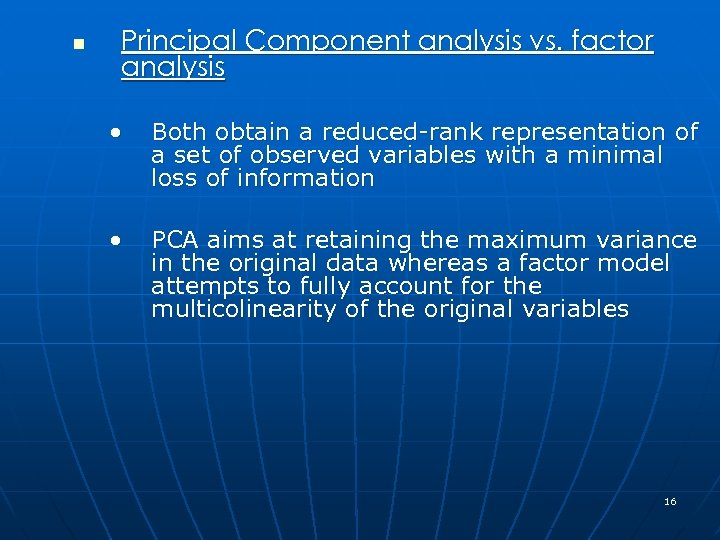n Principal Component analysis vs. factor analysis • Both obtain a reduced-rank representation of a set of observed variables with a minimal loss of information • PCA aims at retaining the maximum variance in the original data whereas a factor model attempts to fully account for the multicolinearity of the original variables 16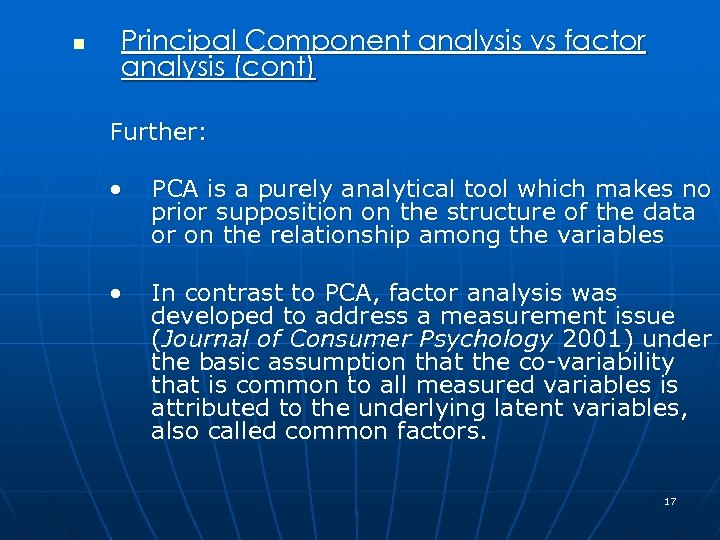n Principal Component analysis vs factor analysis (cont) Further: • PCA is a purely analytical tool which makes no prior supposition on the structure of the data or on the relationship among the variables • In contrast to PCA, factor analysis was developed to address a measurement issue (Journal of Consumer Psychology 2001) under the basic assumption that the co-variability that is common to all measured variables is attributed to the underlying latent variables, also called common factors. 17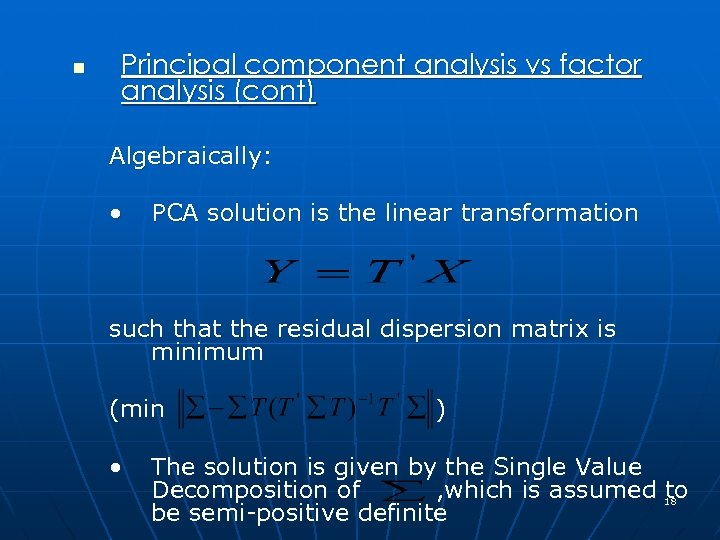n Principal component analysis vs factor analysis (cont) Algebraically: • PCA solution is the linear transformation such that the residual dispersion matrix is minimum (min • ) The solution is given by the Single Value Decomposition of , which is assumed 18 to be semi-positive definite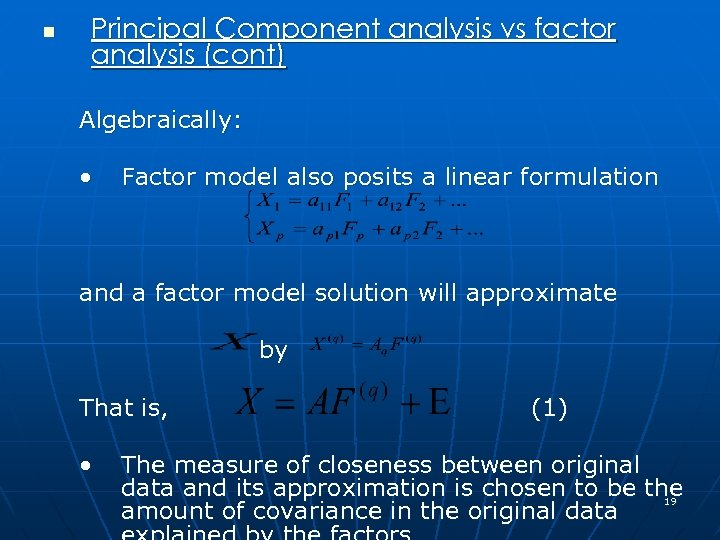n Principal Component analysis vs factor analysis (cont) Algebraically: • Factor model also posits a linear formulation and a factor model solution will approximate by That is, • (1) The measure of closeness between original data and its approximation is chosen to be the 19 amount of covariance in the original data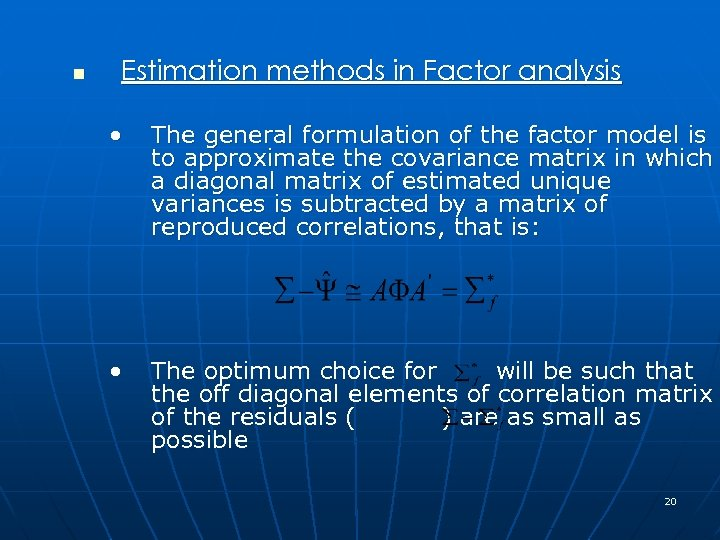n Estimation methods in Factor analysis • The general formulation of the factor model is to approximate the covariance matrix in which a diagonal matrix of estimated unique variances is subtracted by a matrix of reproduced correlations, that is: • The optimum choice for will be such that the off diagonal elements of correlation matrix of the residuals ( ) are as small as possible 20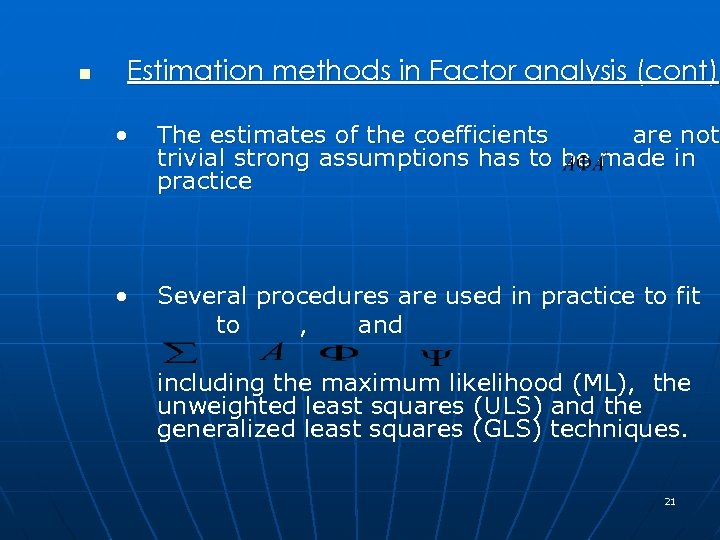n Estimation methods in Factor analysis (cont) • The estimates of the coefficients are not trivial strong assumptions has to be made in practice • Several procedures are used in practice to fit to , and including the maximum likelihood (ML), the unweighted least squares (ULS) and the generalized least squares (GLS) techniques. 21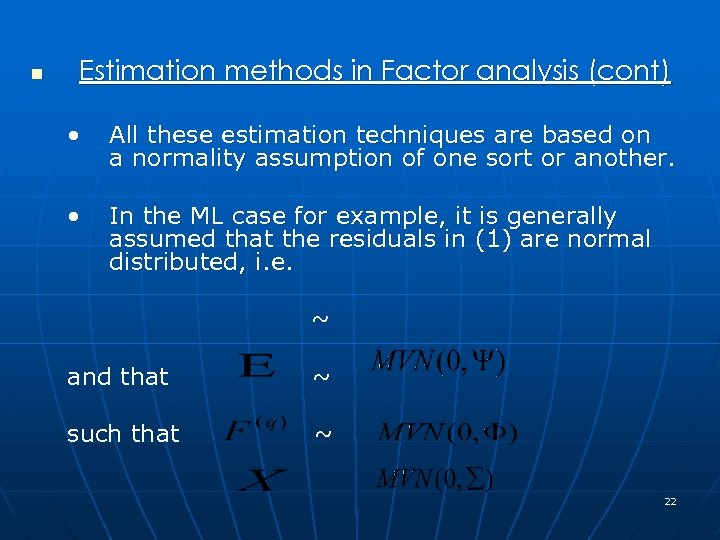n Estimation methods in Factor analysis (cont) • All these estimation techniques are based on a normality assumption of one sort or another. • In the ML case for example, it is generally assumed that the residuals in (1) are normal distributed, i. e. ~ and that ~ such that ~ 22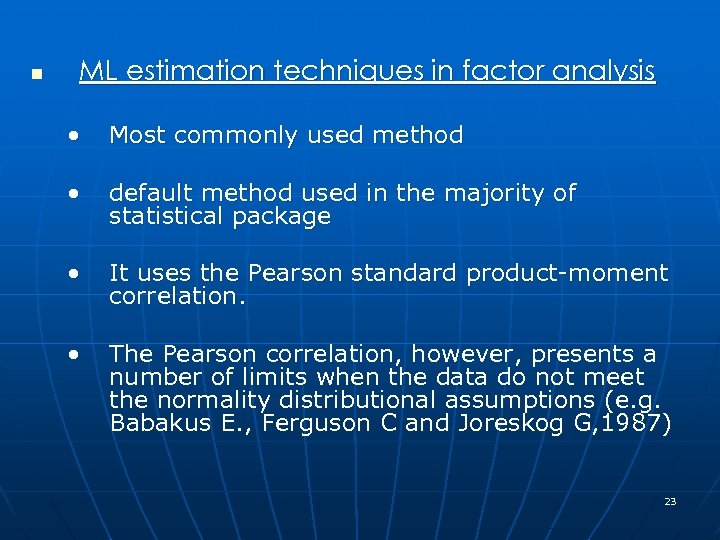n ML estimation techniques in factor analysis • Most commonly used method • default method used in the majority of statistical package • It uses the Pearson standard product-moment correlation. • The Pearson correlation, however, presents a number of limits when the data do not meet the normality distributional assumptions (e. g. Babakus E. , Ferguson C and Joreskog G, 1987) 23n Alternative correlation matrix of categorical ordinal data • Bartelemew 1980, develops an iterative approach to estimate factor scores when the observed variables are dichotomous • Alternative estimation methods for the a common factor model of dichotomous data are discussed in Robert Mislevy (1986) including the Unweighted Least-Squares methods, the Generalized Least Squares solutions and the Maximum Likelihood solutions 24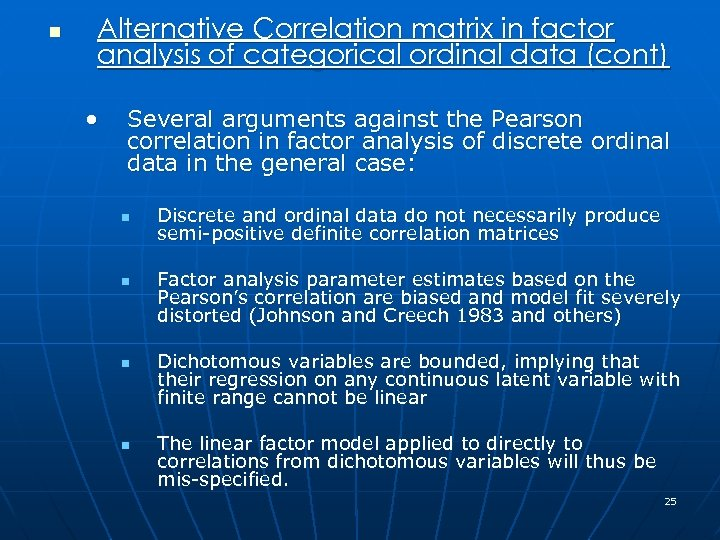n Alternative Correlation matrix in factor analysis of categorical ordinal data (cont) • Several arguments against the Pearson correlation in factor analysis of discrete ordinal data in the general case: n n Discrete and ordinal data do not necessarily produce semi-positive definite correlation matrices Factor analysis parameter estimates based on the Pearson’s correlation are biased and model fit severely distorted (Johnson and Creech 1983 and others) Dichotomous variables are bounded, implying that their regression on any continuous latent variable with finite range cannot be linear The linear factor model applied to directly to correlations from dichotomous variables will thus be mis-specified. 25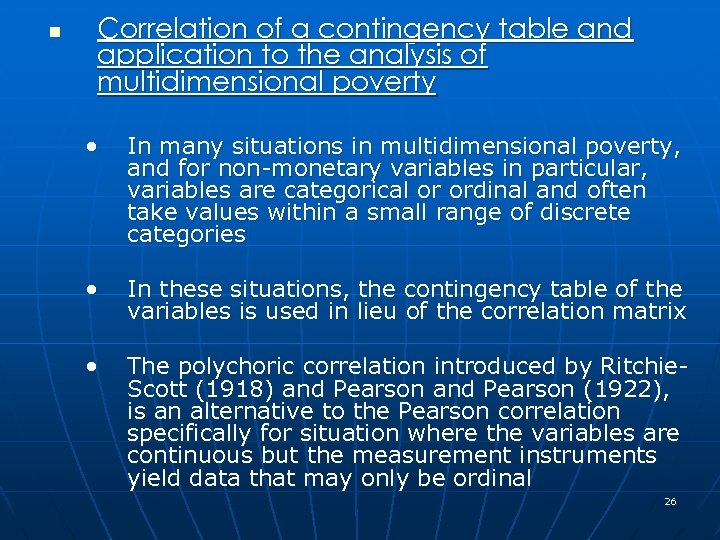n Correlation of a contingency table and application to the analysis of multidimensional poverty • In many situations in multidimensional poverty, and for non-monetary variables in particular, variables are categorical or ordinal and often take values within a small range of discrete categories • In these situations, the contingency table of the variables is used in lieu of the correlation matrix • The polychoric correlation introduced by Ritchie. Scott (1918) and Pearson (1922), is an alternative to the Pearson correlation specifically for situation where the variables are continuous but the measurement instruments yield data that may only be ordinal 26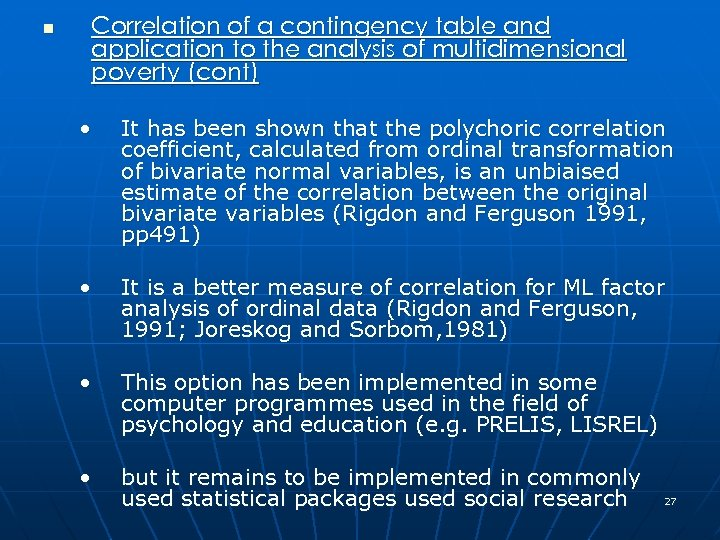n Correlation of a contingency table and application to the analysis of multidimensional poverty (cont) • It has been shown that the polychoric correlation coefficient, calculated from ordinal transformation of bivariate normal variables, is an unbiaised estimate of the correlation between the original bivariate variables (Rigdon and Ferguson 1991, pp 491) • It is a better measure of correlation for ML factor analysis of ordinal data (Rigdon and Ferguson, 1991; Joreskog and Sorbom, 1981) • This option has been implemented in some computer programmes used in the field of psychology and education (e. g. PRELIS, LISREL) • but it remains to be implemented in commonly used statistical packages used social research 27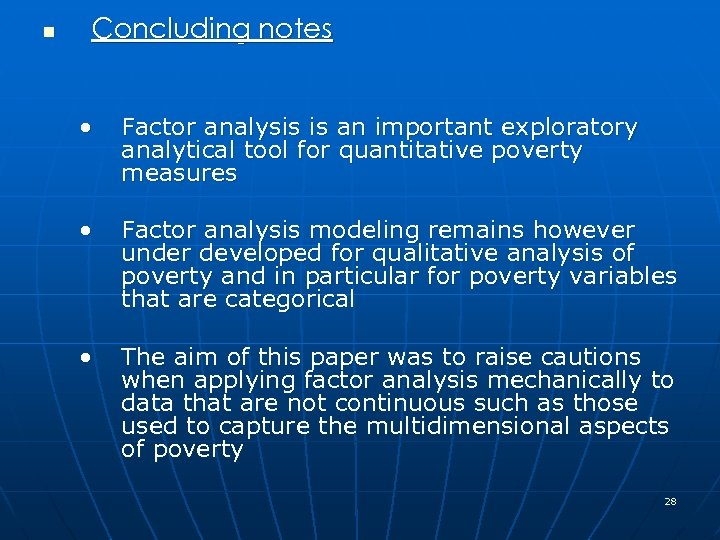n Concluding notes • Factor analysis is an important exploratory analytical tool for quantitative poverty measures • Factor analysis modeling remains however under developed for qualitative analysis of poverty and in particular for poverty variables that are categorical • The aim of this paper was to raise cautions when applying factor analysis mechanically to data that are not continuous such as those used to capture the multidimensional aspects of poverty 28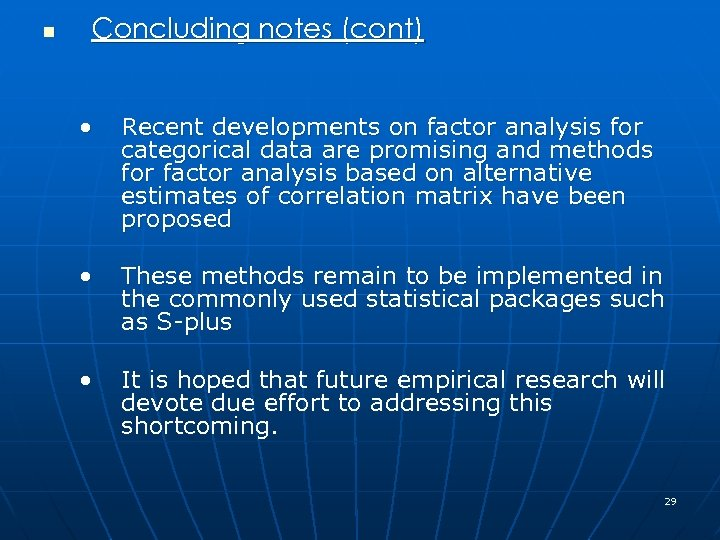n Concluding notes (cont) • Recent developments on factor analysis for categorical data are promising and methods for factor analysis based on alternative estimates of correlation matrix have been proposed • These methods remain to be implemented in the commonly used statistical packages such as S-plus • It is hoped that future empirical research will devote due effort to addressing this shortcoming. 29